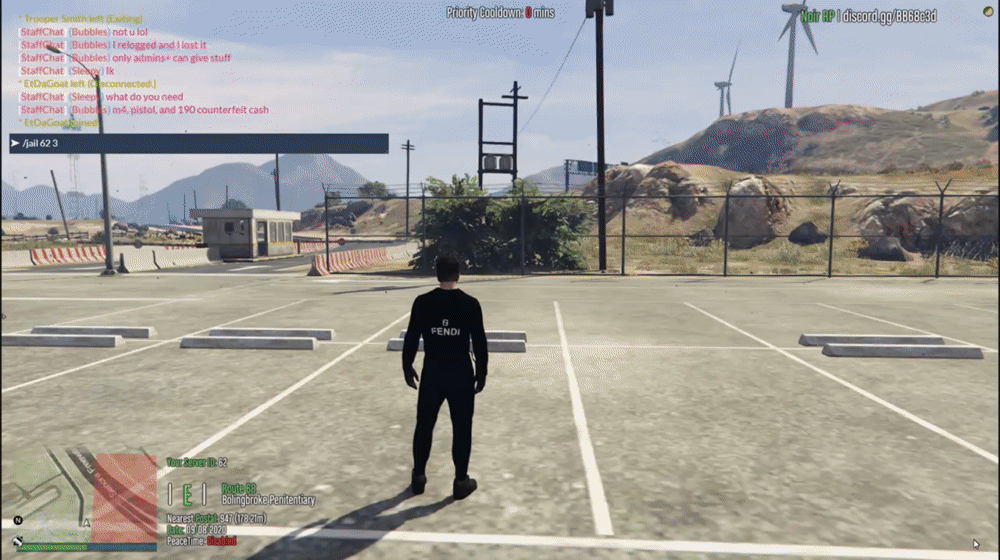### What is it?

Badger_Jailing is a pretty basic jailing script. I wrote it for my past FiveM server because the current jail system was wonky and would let people out of jail if they changed their ped model. This jail script functions a little bit differently than most however. Instead of having a single place to spend jail time, there are cells you define in the config. A player can only be in a cell if it is open. If all the cells are taken, then the player who was jailed will be on a queue waiting to be jailed when a cell is open. In general, I suggest 10+ cells defined in the config as well as a nice jail interior like the one displayed in `Images`. Another nice thing is that jail times carry over even when the server is restarted or a player leaves. Players therefore cannot afford their jail time. You do the crime, you do the time...

### Images### Commands

`/jail <id> <time>` - Requires `Badger_Jailing.Jail` permission.
`/free <id>` - Requires `Badger_Jailing.Unjail` permission.

### Configuration

Config = {
PrisonExit = { x = 1840.57, y = 2586.37, z = 45.95 },
Cells = {
['Cell 1'] = { x = 1774.48, y = 2568.42, z = 49.55 },
['Cell 2'] = { x = 1778.49, y = 2568.26, z = 49.55 },
['Cell 3'] = { x = 1782.50, y = 2568.27, z = 49.55 },
['Cell 4'] = { x = 1786.21, y = 2568.40, z = 49.55 },
['Cell 5'] = { x = 1790.10, y = 2574.27, z = 49.55 },
['Cell 6'] = { x = 1790.03, y = 2577.72, z = 49.55 },
['Cell 7'] = { x = 1790.08, y = 2582.19, z = 49.55 },
['Cell 8'] = { x = 1790.00, y = 2586.09, z = 49.55 },
['Cell 9'] = { x = 1789.73, y = 2590.07, z = 49.55 },
['Cell 10'] = { x = 1789.93, y = 2594.00, z = 49.55 },
['Cell 11'] = { x = 1789.92, y = 2597.65, z = 49.55 },
['Cell 12'] = { x = 1785.60, y = 2602.10, z = 49.55 },
},
}